Huan, Z., Jiao, Z., Li, G., and Wu, X. (2018). "Velocity error correction based tomographic imaging for stress wave nondestructive evaluation of wood," BioRes. 13(2), 2530-2545.

#### Abstract

Stress wave testing has been applied in the nondestructive evaluation of wood for many years. However, the anisotropy property of wood and the limited number of sensors prevent an accurate stress wave velocity measurement and the high resolution of tomographic inversion. This paper proposes a tomographic imaging algorithm (IABLE) with a velocity error correction mechanism. The proposed algorithm computed the wave velocity distribution of the grid cells of wood cross-sections by the least square QR decomposition (LSQR) iterative inversion, and then optimized the tomography with a velocity error correction mechanism (ECM). To evaluate the performance of the proposed algorithm, several healthy and defective logs and live trees were selected as the experimental samples, and the nondestructive testing procedures were finished. With the stress wave velocity data sets measured via a PiCUS 3 stress wave testing instrument, the IABLE algorithm was implemented, and the tomographic images of the log samples and live trees were generated. The experimental results demonstrated the effectiveness of the proposed imaging algorithm for the nondestructive evaluation of wood.

Velocity Error Correction Based Tomographic Imaging for Stress Wave Nondestructive Evaluation of Wood

Zhan Huan,a Zhi Jiao,b Guanghui Li,b,c,* and Xi Wu b

Stress wave testing has been applied in the nondestructive evaluation of wood for many years. However, the anisotropy property of wood and the limited number of sensors prevent an accurate stress wave velocity measurement and the high resolution of tomographic inversion. This paper proposes a tomographic imaging algorithm (IABLE) with a velocity error correction mechanism. The proposed algorithm computed the wave velocity distribution of the grid cells of wood cross-sections by the least square QR decomposition (LSQR) iterative inversion, and then optimized the tomography with a velocity error correction mechanism (ECM). To evaluate the performance of the proposed algorithm, several healthy and defective logs and live trees were selected as the experimental samples, and the nondestructive testing procedures were finished. With the stress wave velocity data sets measured via a PiCUS 3 stress wave testing instrument, the IABLE algorithm was implemented, and the tomographic images of the log samples and live trees were generated. The experimental results demonstrated the effectiveness of the proposed imaging algorithm for the nondestructive evaluation of wood.

Keywords: Nondestructive evaluation of Wood; Stress wave; Tomographic imaging; Error correction

Contact information: a: School of Information Science & Engineering, Changzhou University, 1 Gehu Middle Road, Changzhou 213164,China; b: School of IoT Engineering, Jiangnan University, 1800 Lihu Avenue, Wuxi 214122, China; c: Jiangsu High Technology Research Key Laboratory for Wireless Sensor Networks, 66 Xinmofan Road, Nanjing 210003, China;

* Corresponding author: ghli@jiangnan.edu.cn

INTRODUCTION

The stress wave technique is simple to be applied at a low cost in nondestructive wood evaluation, and it has been used for many years (Wang et al. 2004; Senalik et al. 2014; Carter 2017; Proto et al.2017). Stress wave techniques are simpler and less costly than imaging techniques, and some types of commercial equipment (e.g., Fakopp Treesonic, Fakopp Microsecond, Hitman Resonance Tool, IML Impulse Hammer) are available to measure stress wave transmission times in trees (Wang et al. 2004; Walsh et al. 2014). To increase the reliability of the inspection and decide the extent and location of any internal decay, it would be practical to conduct multiple measurements with more sensors in different orientations at one cross-section, especially for potentially defective trees. Tomographic inversion of stress wave data from multiple measurements allows inspectors to obtain an image of the distribution of stress wave velocity in a cross-section and help estimate the extent of internal decay. Thus, acoustic tomography has been used, with increasing popularity, to assess the internal condition of wood or live trees (Bucur 2003; Socco et al. 2004; Wang et al. 2004, 2009; Dackermann et al. 2014; Senalik et al. 2014; Riggio et al. 2015; Gilbert et al. 2016). Socco et al. (2004) assessed the feasibility of ultrasonic tomography for non-invasive testing of living trees with both laboratory and field ultrasonic measurements. They also pointed out that the marked anisotropy of longitudinal direction properties could create numerous difficulties in constructing 3D tomographic images for trees. Divos and Divos (2005) concluded that the resolution of stress wave-based acoustic tomography is influenced by the applied frequency, the number of sensors, and the applied inversion technique. The resolution of the image increases if the applied frequency is raised, the number of sensors is increased, or the applied algorithm is more advanced. In addition, some sonic tomography equipment (e.g., FAKOPP ArboSonic 3D, PiCUS Sonic Tomograph, and Arbotom) has been developed to conduct multipath stress wave testing, and these products can generate 2D or 3D tomograms of the tree trunk (Arciniegas et al. 2015).

To improve the precision of decay detection and the resolution of tomographic images, many researchers have studied the relationships between the mechanical characteristics of wood and stress wave velocity. Dikrallah et al. (2006) presented an experimental analysis of the acoustic anisotropy of wood, in particular, the dependence of propagation velocities of stress waves on natural anisotropy axis in the cross-section. They found a significant difference in wave velocity between the waves propagating in whole volume and the waves guided on bars. Maurer et al. (2006) developed a simple correction scheme that removes first-order anisotropy effects. The corrected travel-time data can be inverted with isotropic inversion codes that are commercially available. The numerical experiments demonstrate the consequences of ignoring anisotropy effects and the performance of their correction scheme. Liang et al. (2007) found that the prediction of decay areas by acoustic tomography is rather conservative when heartwood decay is the major structural defect in trees. Brancheriau et al. (2008) designed a reconstruction algorithm using a linear approximation of the forward problem (Born approximation) and based on the assumption that a transversal cross-section of wood is isotropic. Qualitative reflectivity images were obtained from back-scattered measurements by reflection tomography. The qualitative aspect of this imaging technique was validated by performing a numerical simulation and testing on a small-diameter green wood (Picea abies) log. Schubert et al. (2009) investigated the influence of fungal decay on tomographic measurements neglecting the heterogeneity of wood, and they found that the cross-section of a cavity, which is larger than 5% of the total cross-section of the trunk, can be detected by acoustic wood tomography. Li et al. (2014) studied stress wave velocity patterns in black cherry trees and found that the ratio of tangential velocity to radial velocity in healthy trees approximated a second-order parabolic curve with respect to the symmetric axis = 0 ( is the angle between wave propagation path and radial direction). The analytical model was found in excellent agreement with the real data from healthy trees. Marin᷉o et al. (2010) analyzed the velocity gradient by relating the value of longitudinal stress-wave velocity to the distance from the point where velocity was measured to the pith, the results of the tests showed a statistically significant relationship between the longitudinal velocity and distance to pith.

Feng et al. (2014) presented an image reconstruction algorithm for detecting the internal defects of wood. The proposed algorithm was based on an interpolation method that estimated the velocity values of unknown grid cells using the values of the surrounding cells. Du et al.(2015) proposed a stress wave tomography method using ellipse-based spatial interpolation and velocity compensation. Their results showed that the proposed method performed well and can resist the signal interference caused by the density variation of the defective area. Arciniegas et al. (2015) used four different signal processing algorithms to study the effect of the signal dynamic on the velocity determination and to determine the validity range of the signal processing techniques. They found that the computed velocity increased as the noise level increased, and they suggested that a combination of the Fisher and Hinkley methods (Fisher 1912; Hinkley 1971) in the same algorithm would yield the most accurate acoustic velocity determinations in the tomography of standing trees. Karlinasari et al. (2016) investigated the sound wave velocity change from green to dry conditions in agarwood and tomographic images associated with changes in the moisture content. The results showed that sonic velocity values increase from 12% to 22% as moisture content decreases from a green condition (live tree) to an air-dried condition (disk sample). Nevertheless, solid and damage areas revealed only slight differences on the tomograms. Gilbert et al.(2016) developed field methodology and image analysis protocols using acoustic tomography to estimate the amount of internal decay and damage of living trees. They concluded that sonic tomography, coupled with image analysis, provides an efficient, noninvasive approach to evaluate decay patterns and the structural integrity of, even irregularly shaped, living trees.

Micro-drilling resistance testing is typically applied to evaluate the internal decay and density variation of wood. A significant amount of research has been conducted to explore the use of resistance drilling measurements for various applications such as tree ring analysis, tree decay detection, and structural timber condition assessment (Faggiano et al. 2011, Wang 2017). From the relative drilling resistance curve, the actual internal decay severity of wood can be decided. Two kinds of micro-drilling testing instruments (Resistograph, Rinntech, Heidelburg, Germany, and IML Resistograph System, IML Inc., Wiesloch, Germany) are often used all over the world. In this study, micro-drilling resistance testing is used to verify the accuracy of tomographic imaging.

The objective of this study is to improve the accuracy of stress wave tomography for the nondestructive evaluation of wood. An error correction mechanism (ECM) of stress wave velocity inversion was proposed for wood tomography reconstruction. Then an imaging algorithm (IABLE) based on the least square QR decomposition (LSQR) and ECM was designed. Experiments were conducted to demonstrate the effectiveness of IABLE.

The Stress Wave Velocity Pattern and Velocity Inversion Procedure

Analytical model of stress wave velocity in healthy live trees

A better understanding of wave velocity patterns in trees is critical to develop reliable and effective imaging software for internal decay detection. For simplicity, one can assume that the trunk of a tree under testing is a cylindrical body, its cross-section is an ideal circularity (Fig. 1(b)), and the pith is located in the center of the circular tree. Figure 1(a) shows the field setup for stress wave testing of a live tree with 12 sensors deployed around the tree trunk. Figure 1(b) gives two stress wave propagation paths, where is a source sensor, and R1 and R2 are receiver sensors. A stress wave is transmitted from the source to the receivers. The SR2 represents the radial direction, and SR1 represents the tangential direction. The VTrepresents the tangential velocity (m/s), VR is the radial velocity (m/s), and is the angle (radian) between radial direction and tangential direction. Generally, the stress wave velocity along radial direction is largest, and it will decrease with the increment of the direction angle . Li et al. (2014) presented an analytical model of the ratio of tangential velocity to radial velocity in sound trees, which approximated a second-order parabolic curve with respect to the symmetric axis = 0 as shown in Eq. 1. They demonstrated that this model was in excellent agreement with the real data measured from healthy trees.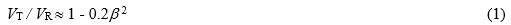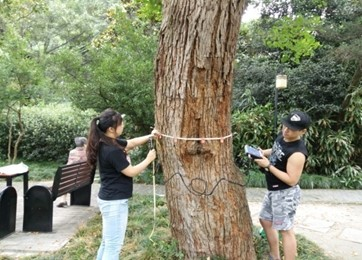(a)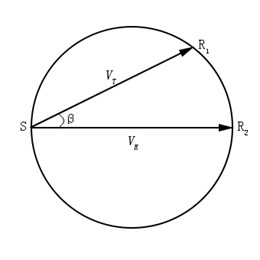(b)

Fig. 1. (a) Field setup of stress wave testing of live trees and (b) stress wave propagation in the cross-section

Given the measured radial wave velocity VR, one can obtain the reference value VT of the tangential wave velocity along any direction in the cross-section from Eq. 1. By comparing the measured velocity Vtest with the reference value Vref, the defective propagation paths can be decided.

Usually, the peripheral reference velocity V0 is determined by calculating the average of the line velocities of the neighboring sensors. This velocity can be used as a reference because the outer part of the tree usually is intact (Divos and Divos 2005). In the second step, one can derive the reference velocity Vref of each path from Eq. 1, and then the measured velocity Vtest is divided by the reference velocity. For each path, if Vtest / Vref < 0.9, then this path will be regarded as a defective line.

Principle of Tomographic Inversion and LSQR Iterative Algorithm

Stress wave tomographic inversion is the procedure that provides the wave velocity distribution in an investigated section (domain) using the impulse travel time and the positions of sensors as inputs. The travel time relative to each SR (source–receiver) position is calculated assuming a certain velocity distribution within the domain, and this velocity distribution is iteratively modified until the calculated travel times are in good agreement with the measured ones (Bucur 2003; Socco et al. 2004; Divos and Divos 2005). Most inversion algorithms for travel-time tomography were developed from seismic investigation (Jackson and Tweeton 1994; Socco et al.2004), and the first step is to split the investigated section into many grid cells of suitable size. For instance, Fig. 2 shows a wave propagation path from S to R, and the wave travel time can be calculated by a line integral along path SR of the wave slowness (reciprocal of velocity) given in Eq. 2.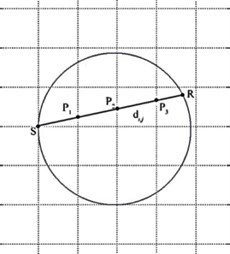Fig. 2. The square grid cells of a cross-section of wood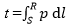(2)

where p is the slowness of stress wave (s/m) and dl is the infinite small distance along the wave path (m).

To reconstruct the slowness distribution, the domain is often divided into M cells, and the continuous function p(x,y) is approximated by the discrete slowness distribution in each cell. Given the assumption that the slowness p is uniform in each cell, Eq. 2 can be transformed into a summation (Socco et al. 2004),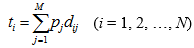(3)

where dij is the segment intercepted by the ith wave path on the jthcell, and pj is the slowness of the jth cell (s/m). Then, the summation can be expressed in matrix form as follows,

T = DP (4)

where T and P are the time (s) and slowness vectors (s/m), respectively, and D is the intercepted segment’s matrix (m).

Given T and matrix D, tomographic imaging process includes solving the slowness vector P, and rendering each cell with appropriate color according to the slowness value. Clearly in Eq. 3, wave path lengths dij are zero for most cells because a given wave path will typically intersect only a few of the cells; thus D is normally a large and sparse matrix. The LSQR iteration algorithm (Paige and Saunders 1982) is used to solve Eq. 4 in this paper. The idea of LSQR iteration algorithm is decomposing the coefficient matrix into an orthogonal matrix Q and an upper triangular matrix R by QR decomposition and then solving the least squares solution iteratively.

Equation 4 is usually an underdetermined equation whose least squares solution is a generalized solution P=P* that makes the residual’s 2-norm (||TDP||2) be a minimum value as follows:

||– DP*||2 = min||– DP||(5)

Thus, the least squares solution of the underdetermined Eq. 4 is equivalent to the solution of the normal Eq. 6:

DTDP = DTT (6)

If (DTD) is reversible, then the normal Eq. 6 has a unique solution. Meanwhile, the least squares solution can be written as:

P = (DTD)-1DTT (7)

The least squares Eq. 7 using the decomposed matrix by QR decomposition can be written as:

P = (RTQTQR)-1(QR)TT = R-1QTT (8)

Then, the least squares solution of the underdetermined equation using QR decomposition is the solution to Eq. 9, whose coefficient matrix is an upper triangular matrix:

RP = QTT (9)

Finally, the solution of the Eq. 9 is used in Eq. 5. The solution is iterated to obtain the least-squares solution until the residual’s 2-norm is minimum (Paige and Saunders 1982).

Imaging Based on Stress Wave Velocity Error Correction

After the velocity inversion procedure, the tomographic image the sample can be generated according to the velocities of the cells. However, the threshold of ratio Vtest Vref (the measured velocity to the reference velocity) is typically an empirical value, which may result in the deviation between the tomography and the actual situation. In this section, the authors proposed a tomographic imaging algorithm based on an ECM. It helps to verify the defective lines; thus the proposed imaging algorithm can improve the quality of tomographic image.

Given a cell and its wave velocity Vc, first the reference velocity Vrefis calculated based on the analytical model (Li et al. 2014). If = Vc Vref < 0.9, then this cell will be decided as defective. Such a simple criterion sometimes results in errors of the generated wood tomography. The defective area often proliferates from one cell to its neighboring cells gradually. There often exists some correlation among the velocities of neighboring cells. In this paper, an ECM was proposed to reduce the potential errors mentioned above. The ECM is a decision strategy based on the stress wave velocities of neighboring cells. For the velocity distribution derived from the LSQR iterative algorithm and a corresponding initial distribution, the authors investigate the velocities of neighboring cells of each cell and adjust the value of ratio Vc / Vref to reduce the probability of decision error. The ECM applies different techniques to deal with different cells of the cross-section of wood. There are two kinds of grid cells, i.e., internal cells and marginal cells. For example, in Fig. 3, the ith cell is an internal cell with four neighboring internal cells, and the jth cell is a marginal cell with only two neighboring internal cells. Here, the cross-section is represented by a circular plane.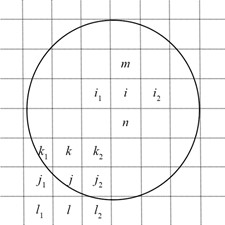Fig. 3. The internal cells and marginal cells of the cross-section

For the internal cells, ECM checks the δ values of the ith cell and its neighboring cells numbered mni1, and i2. If the ratio δ = Vtest / Vrefof each cell has been calculated, the value of δi can be adjusted based on the following criterion,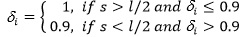(10)

where δi is the ratio Vtest Vref of the ith cell, s is the number of neighboring cells with δ > 0.9, and l is the number of the ith cell’s neighboring cells.

For the marginal cells, such as the jth cell in Fig. 3, the ECM considers its eight neighboring cells (including next nearest neighbors, e.g., k1k2, l1, and l2), and checks which cell is not outside the cross-section. Except for the l1th and lth cells, the other six neighboring cells of jth cell are internal or marginal cells, so ECM uses the δ values of these six cells to correct the value of δj based on Eq. 10.

When all cells have been investigated with the aforementioned procedure, it should be checked whether the resulted distribution has been changed compared with the initial δ distribution. If there is any change in δ distribution, the ECM needs to repeat the procedure until the distribution remains stable. Once the final δ distribution is decided, each cell of the cross-section will be marked with appropriate color based on its δ value, and then the tomographic image can be generated.

Experimental

Materials

Two experiments were conducted with logs and live trees as the samples. The first experiment used three log samples (#1, #2, and #3) from laboratory including a defective cedar log with an artificial cavity, a defective sapium log, and a sound cedar log. The second experiment was finished in September 2016, three kinds of live trees with internal defects (Juniperus chinensisLiquidambar formosana, and Morus alba) of Slender West Lake Park, Yangzhou, China, were selected as samples (#4, #5, and #6).

Methods

In the experiments, a PiCUS 3 Sonic Tomograph (Argus Electronic Gmbh, Rostock, Germany) instrument was used to collect the stress wave velocities and tomographic images. For each tested sample, 10 or 12 sensors were deployed according to the size of its cross-sections. In addition, a Resistograph microdrilling instrument (RINNTECH, Heidelberg, Germany) was used to evaluate the defective degree of the cross-sections and demonstrate the accuracy of the proposed imaging algorithm. For the experiment of live trees, three live trees were tested using the PiCUS instrument, each at different heights above the ground. The tomographic images were then generated by IABLE and PiCUS. Subsequently, the samples were tested using the Resistograph microdrilling instrument at the same location as the PiCUS to measure the defective degree of the cross-sections. The Resistograph was accurate in defining the actual length of defective segment.

RESULTS AND DISCUSSION

Experimental Results of Logs

Three log samples were tested using the PiCUS Sonic Tomograph instrument. The proposed tomographic imaging algorithm was implemented based on the velocity data set collected by the PiCUS. Then, the authors compared the tomographic images generated by IABLE and PiCUS with the actual situation of the sample. The ratio (Rs) of the defective area to the whole cross-section area reflects the accuracy of images, which was calculated by dividing the number of pixels in the defective area by those of the cross-section. Let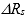(%) represent the relative error between the Rs calculated from the tomographic image of IABLE or PiCUS and the actual Rs of the sample, which is defined by Eq. 11,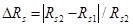(11)

where Rs1 (%) is the Rs of IABLE or PiCUS and Rs2 (%) is the actual Rs.

Figures 4 through 6 show the log samples and their tomographic images generated by IABLE and PiCUS. Here, for the images of IABLE, the brown color represents the defective area, and the green, purple, and blue colors were the defective area for PiCUS.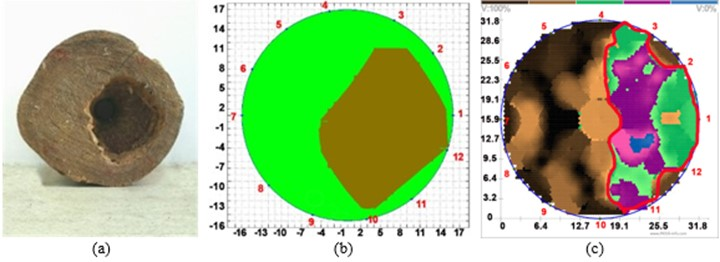Fig. 4. (a) Sample #1, (b) tomographic image of IABLE, and (c) tomographic image of PiCUS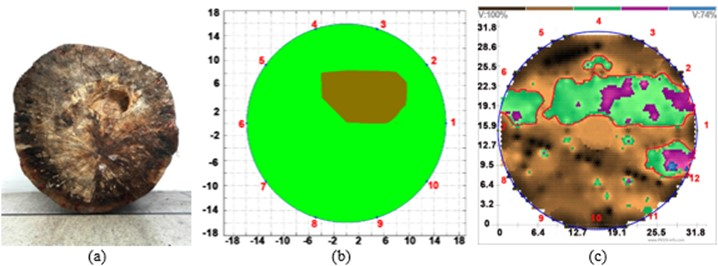Fig. 5. (a) Sample #2, (b) tomographic image of IABLE, and (c) Tomographic image of PiCUS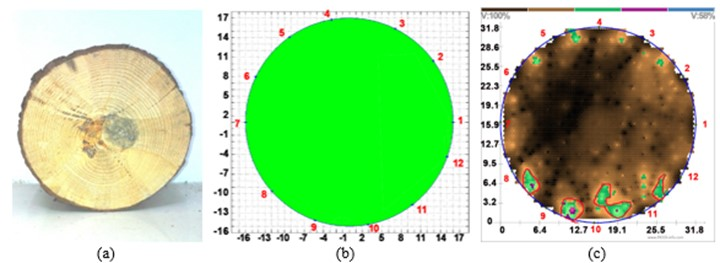Fig. 6. (a) Sample #3, (b) tomographic image of IABLE, and (c) tomographic image of PiCUS

Table 1 shows the Rs of images generated by IABLE and PiCUS. Here,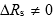meant that the tomographic image displayed a false positive or false negative for the defective area. A low moisture content in the log samples caused false defective areas. From Table 1, it can be seen that the Rs of IABLE was closer to the actual Rs and its relative error was smaller than that of PiCUS. However, it was difficult to eliminate the false defects thoroughly in either method (as shown in Fig. 5).

Table 1. Imaging Accuracy Comparison Between IABLE and PiCUS

##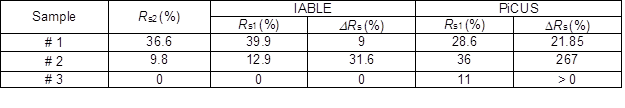Experimental Results of Live Trees

Figures 7, 9, and 11 depict the sample trees under test and the tomographic images generated by IABLE and PiCUS of the tree trunk. Figures 8, 10, and 12 give the resistance curves of the Resistograph along different testing paths, where the horizontal axis denotes the length of the testing path and the blue section is the actual length without bark. The longitudinal axis represents the relative resistance. In each resistance curve, the path ij accorded with the wave path in the tomographic image of the cross-section from the ithsensor to jth sensor.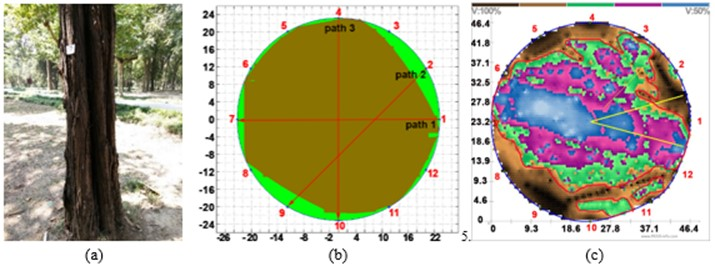Fig. 7. (a) Sample #4, (b) tomographic image of IABLE, and (c) tomographic image of PICUS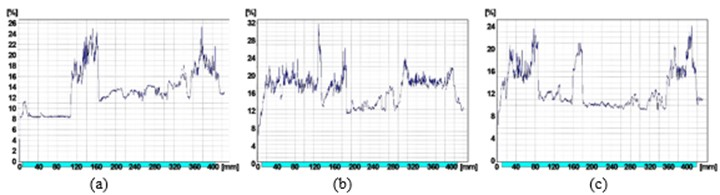Fig. 8. (a) Resistance curve of path 1 through 7; (b) Resistance curve of path 2 through 9; and (c) Resistance curve of path 4 through 10

From Fig. 7, it can be seen that the defective area of the tomographic image of IABLE was very close to that of PiCUS. To compare the accuracy of the defective areas of IABLE and PiCUS, the drilling resistance testing processes along three different paths were completed. Figure 8 shows the resistance curves of three paths. In the resistance curves, the horizontal axis denotes the drilling depth (mm), and the vertical axis represents the relative drilling resistance Resi(%). Although both IABLE and PiCUS showed a high accuracy of defect detection, there was a slight decay that was found by IABLE but not by PiCUS at the beginning of path 1 through 7. Along path 2 through 9, except for the obvious defect at 190 mm to 310 mm, there was a slight internal decay in the sample #4 tree, and IABLE obtained a better evaluation than PiCUS. The same case was found in path 4 through 10.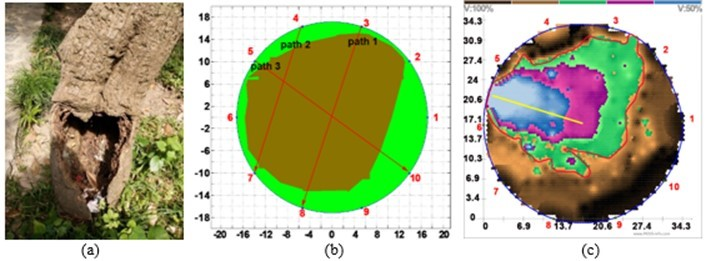Fig. 9. (a) Sample #5, (b) tomographic image of IABLE, and (c)tomographic image of PiCUS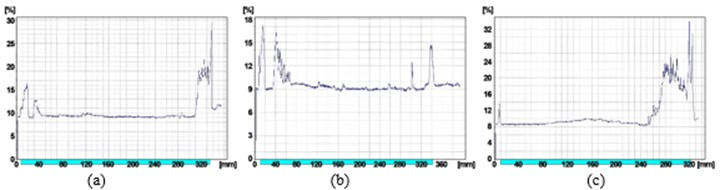Fig. 10. (a) Resistance curve of path 3 through 8; (b) Resistance curve of path 4 through 7; and (c) Resistance curve of path 5 through 10

Figure 9 shows the sample #5 tree with a large cavity and the tomographic images generated by IABLE and PiCUS, and Fig. 10 shows the resistance curves of Resistograph along three paths. Due to the large cavity, the tomographic image generated by PiCUS was not accurate enough. Instead, IABLE corrected the error caused by the abnormal data with ECM and made the image reflect the cavity more accurately.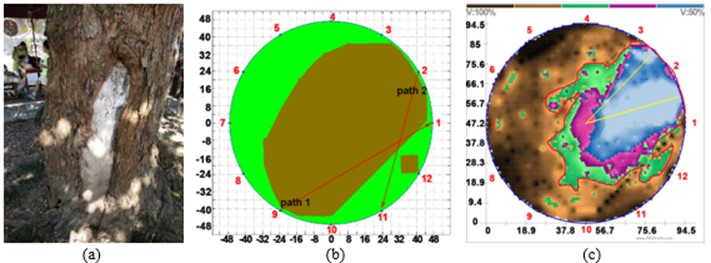Fig. 11. (a) Sample #6, (b) tomographic image of IABLE, and (c) tomographic image of PiCUS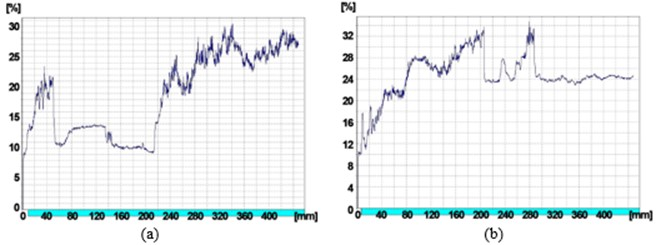Fig. 12. (a) Resistance curve of path 9 through 1 and (b) Resistance curve of path 2 through 11

From Fig. 11, it can be seen that there was a clear cavity in the sample #6 tree, and it was found that the cavity was filled with special padding, which made the defective areas have a high Resi value. Figure 11 shows the sample tree and the tomographic images generated by IABLE and PiCUS. Figure 12 presents the resistance curves along two paths. The radius of tree trunk was more than the drilling range of Resistograph instrument, so the authors could only obtain the Resi data of half path. As shown in Fig. 12(a), the Resi values were relatively low around the sensor #9, so this section can be regarded as defective, and this defect line was detected by IABLE clearly. However, PiCUS was unable to detect this defect. Therefore, from Fig. 11, it can be seen that the tomographic image of IABLE displayed some small defective area disconnected with the central defective area that should be improved in the future.

To quantitatively evaluate the performance of IABLE, for the same stress wave propagation path, the ratio (Rl) of the defective length calculated from the image of IABLE or PiCUS to the actual defective length tested by the Resistograph instrument was compared. Like the definition of RsRl represents the relative error between the Rl of IABLE or PiCUS and the actual Rl. Table 2 gives the comparison results of IABLE and PiCUS for the three live tree samples. Here, Rl1and Rl1 are the above-defined ratios of IABLE and PiCUS, respectively. This experiment showed that IABLE could detect the slight decay, which was negated by PiCUS. For samples #4 and #5, IABLE had Rl values less than 10%, which showed a better evaluation for the slight decay than PiCUS. For sample #6, due to the incomplete data of microdrilling testing, there existed differences between the tomographic images and REL data of resistance curves. In general, it can be seen that IABLE had higher accuracy than PiCUS, and better reflects the actual situation. However, the boundary of the defective area in the image generated by IABLE was not realistic enough, which requires a more effective strategy of velocity error correction for the marginal cells.

Table 2. The Length Ratio and Relative Error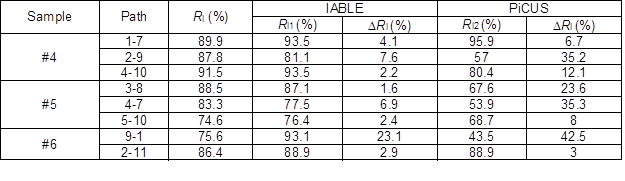Conclusions

Traditionally, tomographic imaging for stress wave testing of wood is based on velocity inversion. However, the anisotropy property of wood and the limited number of sensors prevent an accurate stress wave velocity measurement and high resolution of tomographic inversion. This study presented a velocity error correction mechanism for improving the accuracy of tomographic imaging of wood. Based on the preliminary results obtained from both laboratory and field-testing, the following conclusions were drawn:

1. The velocity error generated during the inversion procedure was corrected by the collaboration of neighboring grid cells, effectively improving the accuracy of tomography.
2. The experimental results of several samples of logs and live trees showed that the IABLE tomographic imaging algorithm developed in this work had better accuracy than the PiCUS instrument. The quantitative comparison between the microdrilling resistance testing and the stress wave tomographic image demonstrated the effectiveness of IABLE.
3. The experiments showed that the tomographic images of IABLE were not precise enough for the small defects and boundary shape. Thus, the proposed velocity error correction mechanism needs improvement.
4. Micro-drilling testing can help people demonstrate the accuracy of acoustic imaging of wood, and develop imaging equipment with high resolution for nondestructive evaluation wood.

ACKNOWLEDGMENTS

This study was supported in part by the National Natural Science Foundation of China (Grant No. 61472368 and 61772248), the Key Research and Development Project of Jiangsu Province (Grant No. BE2016627), the Fundamental Research Funds for the Central Universities (Grant No. RP51635B), the Wuxi International Science and Technology Research and Development Project (Grant No. CZE02H1706), and the Jiangsu Innovation Project of Postgraduate Training (Grant No. SJLX16_0499).

REFERENCES CITED

Arciniegas, A., Brancheriau, L., and Lasaygues, P. (2015). “Tomography in standing trees: Revisiting the determination of acoustic wave velocity,” Annals of Forest Science72(6), 685-691. DOI: 10.1007/s13595-014-0416-y

Brancheriau, L., Lasaygues, P., Debieu, E., and Lefebvre, J. (2008). “Ultrasonic tomography of green wood using a non-parametric imaging algorithm with reflected waves,” Annuals of Forest Science 65(7), 712-717. DOI: 10.1051/forest:200851

Bucur, V. (2003). Nondestructive Characterization and Imaging of Wood, Springer-Verlag, Berlin, Germany.

Carter, P. (2017). “Acoustic Technology—Enhanced Tools for Research and Operations,” in: Proceedings of the 20th International Symposium on Nondestructive Testing of Wood, Madison, WI, USA, pp. 58-70.

Dackermann, U., Crews, K., Kasal, B., Li, J., Riggio, M., Rinn, F., and Tannert, T. (2014). “In situ assessment of structural timber using stress-wave measurements,” Materials and Structures47(5), 787-803. DOI: 10.1617/s11527-013-0095-4

Dikrallah, A., Hakam, A., Kabouchi, B., Brancheriau, L., Bailleres, H., Famiri, A., and Ziani, M. (2006). “Experimental analysis of acoustic anisotropy of green wood by using guided waves,” ESWM-COST Action 35, 149-154. DOI: 10.13140/2.1.2936.1600

Divos, F., and Divos, P. (2005). “Resolution of stress wave based acoustic tomography,” in: Proceedings of the 14th International Symposium on Nondestructive Testing of Wood, Shaker Verlag, Eberswalde, Germany, pp. 309-314.

Du, X., Li, S., Li, G., Feng, H., and Chen, S. (2015). “Stress wave tomography of wood internal defects using ellipse-based spatial interpolation and velocity compensation,” BioResources 10(3), 3948-3962. DOI: 10.15376/biores.10.3.3948-3962

Faggiano, B., Grippa, M. R., Marzo, A., and Mazzolani, F. M. (2011). “Experimental study for non-destructive mechanical evaluation of ancient chestnut timber,” Journal of Civil Structure Health Monitoring1, 103-112. DOI: 10.1007/s13349-011-0011-y. (Italy)

Feng, H., Li, G., Fu, S., and Wang, X. (2014). “Tomographic image reconstruction using an interpolation method for tree decay detection,” BioResources 9(2), 3248-3263. DOI: 10.15376/biores.3248-3263

Fisher, R. A. (1912). “On an absolute criterion for fitting frequency curves,” Messenger of Mathematics 41, 155-160

Gilbert, G. S., Ballesteros, J. O., Barrios-Rodriguez, C. A., Bonadies, E. F., Cedeño-Sánchez, M. L., Fossatti-Caballero, N. J., Trejos-Rodríguez, M. M., Pérez-Suñiga, J. M., Holub-Young, K. S., Henn, L., et al. (2016). “Use of sonic tomography to detect and quantify wood decay in living trees,” Applications in Plant Sciences 4(12), Article ID1600060. DOI: 10.3732/apps.1600060

Hinkley, D. V. (1971). “Inference about the change-point from cumulative sum tests,” Biometrika 58(3), 509-523. DOI: 10.1093/biomet/58.3.509

Jackson, M., and Tweeton, D. (1994). Migratom – Geophysical Tomography Using Wavefront Migration and Fuzzy Constraints (Report of Investigation: RI 9497), U. S. Department of the Interior, Washington D.C., USA.

Karlinasari, L., Putri, N., Turjaman, M., Wahyudi, I., and Nandika, D. (2016). “Moisture content effect on sound wave velocity and acoustic tomograms in agarwood trees (Aquilaria malaccensis Lamk.),” Turkish Journal of Agriculture and Forestry 40, 696-704. DOI: 10.3906/tar-1511-74

Li, G., Wang, X., Feng, H., Wiedenbeck, J., and Ross, R. J. (2014). “Analysis of wave velocity patterns in black cherry trees and its effect on internal decay detection,” Computers and Electronics in Agriculture 104(6), 32 -39. DOI: 10.1016/j.compag.2014.03.008

Liang, S., Wang, X., Wiedenbeck, J., Cai, Z., and Fu, F. (2007). “Evaluation of acoustic tomography for tree decay detection,” in: Proceedings of the 15th International Symposium on Nondestructive Testing of Wood, Eberswalde, Germany, pp. 49-54.

Marin᷉o, R. A., Fernández, M. E., Fernández-Rodríguez, C., and Méndez, M. (2010). “Detection of pith location in chestnut lumber (Castanea sativa Mill.) by means of acoustic tomography and longitudinal stress-wave velocity,” European Journal of Wood and Wood Products 68(2), 197–206. DOI: 10.1007/s00107-009-0366-5

Maurer, H., Schubert, S., Bachle, F., Clauss, S., Gsell, D., Dual, J., and Niemz, P. (2006). “A simple anisotropy correction procedure for acoustic wood tomography,” Holzforschung 60(5), 567-573. DOI: 10.1515/HF.2006.094

Paige, C., and Saunders, M. (1982). “LSQR: An algorithm for sparse linear equations and sparse least squares,” ACM TOMS 8(1), 43-71. DOI: 10.1145/355984.355989

Proto, A.R., Macrì, G., Bernardini, V., Russo, D., and Zimbalatti, G. (2017). “Acoustic evaluation of wood quality with a non-destructive method in standing trees: A first survey in Italy,” iForest 10, 700-706. DOI: 10.3832/ifor2065-010

Riggio, M., Sandak, J., and Franke, S. (2015). “Application of imaging techniques for detection of defects, damage and decay in timber structures on-site,” Construction and Building Materials 101(Part 2), 1241-1252. DOI: 10.1016/j.conbuildmat.2015.06.065

Schubert, S., Gsell, D., Dual, J., Motavalli, M., and Niemz, P. (2009). “Acoustic wood tomography on trees and the challenge of wood heterogeneity,” Holzforschung 63(1), 107-112. DOI: 10.1515/HF.2009.028

Senalik, C.A., Schueneman, G., and Ross, R. J. (2014). Ultrasonic-based Nondestructive Evaluation Methods for Wood: A Primer and Historical Review. General Technical Report (FPL-GTR-235), USDA, Forest Service, Forest Products Laboratory, Madison, WI.

Socco, L., Sambuelli, L., Martinis, R., Comino, E., and Nicolotti, G. (2004). “Feasibility of ultrasonic tomography for nondestructive testing of decay on living trees,” Research in Nondestructive Evaluation 15(1), 31-54. DOI: 10.1080/09349840490432678

Walsh, D., Strandgard, M., and Carter, P. (2014) . “Evaluation of the Hitman PH330 acoustic assessment system for harvesters,” Scandinavian Journal of Forest Research. 29(6), 593-602, DOI: 10.1080/02827581.2014.953198.

Wang, X., Divos, F., Pilon, C., Brashaw, B. K., Ross, R. J., and Pellerin, R. F. (2004). Assessment of Decay in Standing Timber Using Stress Wave Timing Nondestructive Evaluation Tools, A Guide for Use and Interpretation (FPL-GTR-147), USDA, Forest Service, Forest Products Laboratory, Madison, WI.

Wang, X., Wiedenbeck, J., and Liang, S. (2009). “Acoustic tomography for decay detection in black cherry trees,” Wood and Fiber Science 41(2), 127-137. DOI: 10.1.1.585.1668

Wang, X. (2017). “Partial resistance drilling to assess wood density in trees,” in: Proceedings of the 20th International Symposium on Nondestructive Testing of Wood, Madison, WI, USA, pp. 22-32.

Article submitted: November 30, 2017; Peer review completed: February 2, 2018; Revisions accepted: February 12, 2018; Published: February 14, 2018.

DOI: 10.15376/biores.13.2.2530-2545